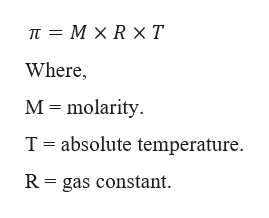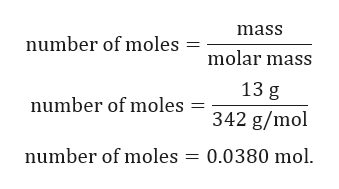27) A solution is made by dissolving 13 g of sucrose, C12H22O11, in 117 g of water, producing asolution with a volume of 125 mL at 20°C. What is the expected osmotic pressure at 20°C? A)10 atm   B)14 atm     C)58 atm     D)7.3 atm

Question

27) A solution is made by dissolving 13 g of sucrose, C12H22O11, in 117 g of water, producing asolution with a volume of 125 mL at 20°C. What is the expected osmotic pressure at 20°C?

A)10 atm   B)14 atm     C)58 atm     D)7.3 atm

Step 1

Given:

Mass of sucrose = 13 g.

Volume of water = 117 mL = 0.117 L.

Molar mass of sucrose = 342 g/mol.

Volume of solution = 117 mL = 0.117 L.

Temperature T = 20℃ = 20+273K = 293K.

Step 2

Osmotic pressure can be calculated as:help_outlineImage Transcriptioncloseп 3 МXRхТ Where, M molarity T absolute temperature R gas constant fullscreen
Step 3

Number of moles of solute...help_outlineImage Transcriptionclosemass number of moles molar mass 13 g number of moles= 342 g/mol number of moles 0.0380 mol. fullscreen

Want to see the full answer?

See Solution

Want to see this answer and more?

Our solutions are written by experts, many with advanced degrees, and available 24/7

See Solution
Tagged in

Solutions